﻿ Reference > Transforms > Calculate

# Calculate

## Description

Performs a calculation on 1 or 2 columns and creates a new column.

## Examples

Round a column of numbers to the nearest integer: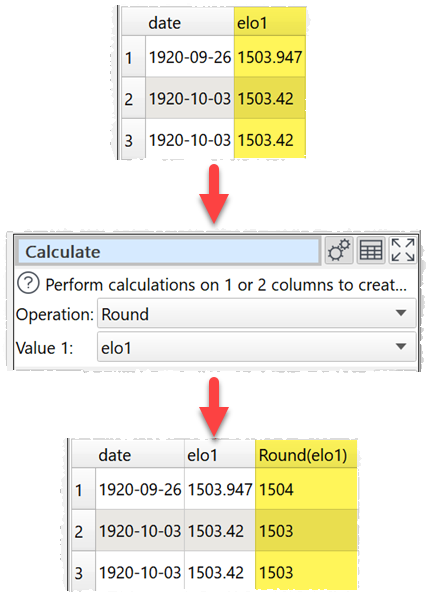Divide a column of numbers by 100: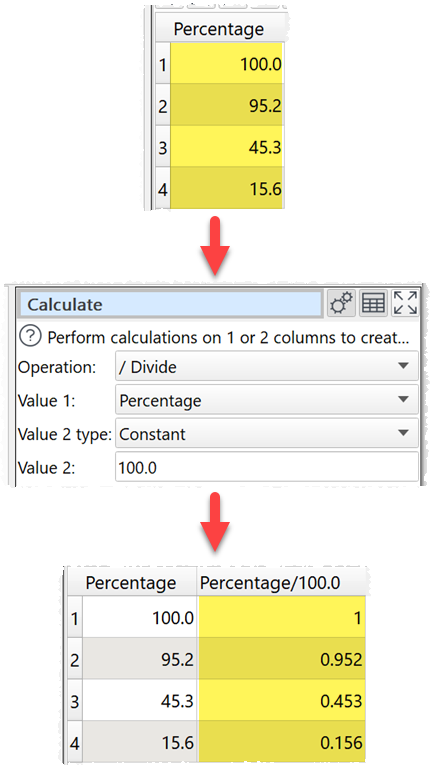Multiply 2 columns of numeric values: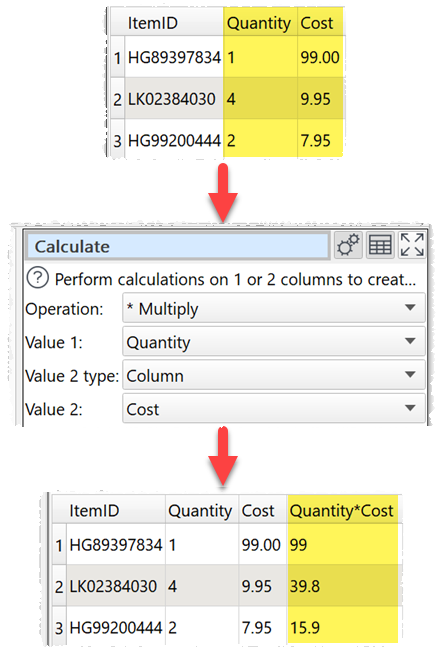Add a days column to a date column: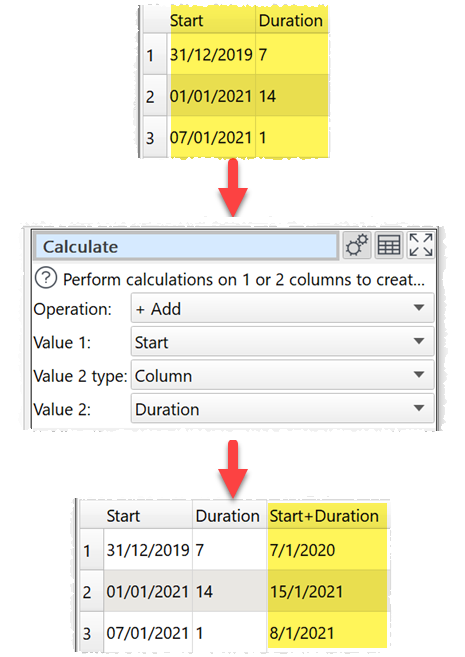Calculate the difference in days between 2 date columns: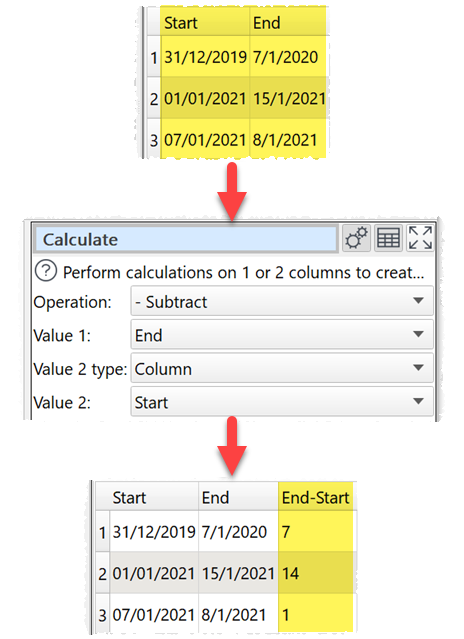One.

## Options

Set Operation to the operation you wish to perform.

Set Value 1 to the first column of values you wish to operate on.

Set Value 2 type depending on whether you wish to use a single value or a column of values for the second value (binary operations only).

Set Value 2  to the second column of values or constant value you wish to operate on (binary operations only).

## Notes

The following operations are supported.

Operation

Value 1

Value 2

Notes

Examples

Number

Number

1.2 + 3 = 4.2

Date

Integer number

31/01/2021 + 7 = 07/02/2021

- Subtract

Number

Number

Numerical subtraction.

1.2 - 3 = -1.8

Date

Integer number

Subtracts days from the date.

31/01/2021 - 7 = 24/01/2021

Date

Date

Days the date in Value 1 is after the date in Value 2 (negative if before).

31/01/2021 - 24/01/2021 = 7

24/01/2021 - 31/01/2021 = -7

* Multiply

Number

Number

Numerical multiplication.

1.2 * 3 = 3.6

/ Divide

Number

Number

Numerical division.

1.2 / 3 = 0.4

1 / 0 = Error

^ Power

Number

Number

Column 1 to the power Column 2 (col1col2).

1.2 ^ 3 = 1.728

2 ^ -3 = 0.125

% Modulus

Integer number

Integer number

Remainder after Value 1 is divided by Value 2.

7 % 2 = 1

7 % 0 = Error

Abs

Number

N/A

The absolute value.

Abs( 1.2 ) = 1.2

Abs( -1.2 ) = 1.2

And

Boolean

Boolean

Logical AND.

And( true, false ) = false

And( 1, 1 ) = true

And( 0, FALSE ) = false

Ceiling

Number

N/A

The smallest integer that is not less than the value.

Ceiling( 1 ) = 1

Ceiling( 1.2 ) = 2

Ceiling( -1.2 ) = -1

DateTimeToMSecs

ISO datetime

Integer number

Convert an ISO datetime to the number of milliseconds since 1970-01-01:00:00:00.000 UTC .

DateTimeToMSecs( 1970-01-01 ) = 0

DateTimeToMSecs( 2022-09-15T21:06:10 ) = 1663272370000

DateTimeToMSecs( 1969-12-30T13:42:23.211Z ) = -123456789

DateTimeToMSecs( 2022-09-16T13:00:02+01:00 ) = 1663329602000

DayOfWeek

Date

N/A

Day of the week, from 1 = Monday to 7 = Sunday.

DayOfWeek( 31/01/2021 ) = 7

DayOfWeek( 01/02/2021 ) = 1

DayOfMonth

Date

N/A

Day of the month, from 1 to 31.

DayOfMonth( 31/01/2021 ) = 31

DayOfMonth( 01/02/2021 ) = 1

DayOfYear

Date

N/A

Day of the year, from 1 to 366.

DayOfYear( 01/02/2021 ) = 32

DayOfYear( 31/12/2021 ) = 365

Decrement

Number

N/A

Subtract 1.

Decrement( 1 ) = 0

Decrement( -1 ) = -2

Decrement( 1.2 ) = 0.2

Date

N/A

Subtract 1 day.

Decrement( 31/01/2021 ) = 30/01/2021

Floor

Number

N/A

The largest integer that is not greater than the value.

Floor( 1 ) = 1

Floor( 1.2 ) = 1

Floor( -1.2 ) = -2

Increment

Number

N/A

Increment( 1 ) = 2

Increment( -1 ) = 0

Increment( 1.2 ) = 2.2

Date

N/A

Increment( 31/01/2021 ) = 01/02/2021

JulianDay

Date

N/A

Days since the beginning of the Julian period.

JulianDay( 31/01/2021 ) = 2459246

JulianDay( 01/02/2021 ) = 2459247

Length

Any

N/A

Then number of characters in the value (include any whitespace).

Length( 1.2 ) = 3

Length( 31/01/2021 ) = 10

Length( abcd ) = 4

LevDistance

Any

Any

Calculates the Levenshtein distance between 2 text values. Case sensitive.

LevDistance( Easy Data, easydata ) = 3

Ln

Number

N/A

The natural (base e) logarithm of the value.

Ln ( 10 ) = 2.302

Ln ( 0 ) = Error

Maximum

Number

Number

Returns the larger number.

Maximum( 10, 11 ) = 11

Maximum( 10, -11 ) = 10

Date

Date

Returns the later date.

Maximum( 31/01/2021, 01/01/2022 ) = 01/01/2022

Minimum

Number

Number

Returns the smaller number.

Minimum( 10, 11 ) = 10

Minimum( 10, -11 ) = -11

Date

Date

Returns the earlier date.

Minimum( 31/01/2021, 01/01/2021 ) = 31/01/2021

MSecsToDateTime

Integer number

ISO datetime

Convert the number of milliseconds since 1970-01-01:00:00:00.000 UTC to an ISO datetime.

MSecsToDateTime( 0 ) = 1970-01-01T00:00:00.000

MSecsToDateTime( -123456789 ) = 1969-12-30T13:42:23.211

MSecsToDateTime( 123456789 ) = 1970-01-02T10:17:36.789

Log10

Number

N/A

The common (base 10) logarithm of the value.

Log10( 10 ) = 1

Log10( 0 ) = Error

Month

Date

N/A

1 = January.

Month( 31/12/2021 ) = 12

Or

Boolean

Boolean

Logical OR.

Or( true, false ) = true

Or( 1, 1 ) = true

Or( 0, FALSE ) = false

Round

Number

N/A

Round to the nearest integer.

Round( 1 ) = 1

Round( -1.2 ) = -1

Round( 1.5 ) = 2

Sign

Number

N/A

1 if value > 0, 0 if value = 0, -1 of value < 0.

Sign( 1.2 ) = 1

Sign( 0 ) = 0

Sign( -1.2 ) = -1

WeekOfYear

Date

N/A

The year and the week of the year, from 01 to 53, separated with a comma. In accordance with ISO 8601, each week falls in the year to which most of its days belong, in the Gregorian calendar. As ISO 8601's week starts on Monday, this is the year in which the week's Thursday falls.

WeekOfYear( 31/12/2020 ) = 2020,53

WeekOfYear( 06/01/2021 ) = 2021,01

WeekOfYear( 31/12/2021 ) = 2021,52

Xor

Boolean

Boolean

Logical XOR (exclusive OR).

Xor( true, false ) = true

Xor( 1, 1 ) = false

Xor( 0, FALSE ) = false

Year

Date

N/A

The 4 digit year.

Year( 31/01/2021 ) = 2021

Each row is calculated separately.

Whether values are interpreted as number, dates or text depends on Supported date formats and locale.

To concatenate text in different columns use the Concat Cols transform.

To compare 2 columns use the Compare Cols transform.

For logical operations use the If transform.

For operations on whole rows or columns use the Stats transform.

For more complex number/date calculations use the Javascript transform.

To modify the numerical precision of the results use the Num Format transform.

To add a new column of values use the New Col transform.